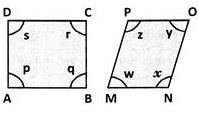Courses

# ABCD and MNOP are quadrilaterals as shown in the figure.Which of the following is correct?a)p+q+r+s = w+x+y+zb)p+q+r+s < w+x+y+zc)p+q+r+s > w+x+y+zd)Either (B) or (C)Correct answer is option 'A'. Can you explain this answer? Related Test: Test: Understanding Quadrilaterals- 3

## Class 8 Question

By Rabindra Jha · Aug 08, 2020 ·Class 8
Kritika I Bat Ani answered Aug 08, 2020
Yes , the correct answer is A . ABCD is a Sruare and MNOP is Parallelogram .[ The angles in Square named as PQRS and in Parallelogram , angles named as XYWZ] . answer is a because Square has all the properties of Parellelogram.